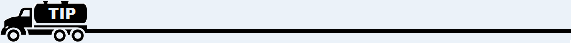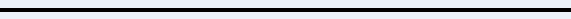﻿ COGS for Small Items

# Cost of Goods Sold for Small Items

At the end of each period (month), calculate the Cost Of Goods Sold for those items under \$100 (such as fittings or black pipe). There may well be many different costs for this kind of item. However, it is common practice that a consistent markup is used.

If this is the case, use the following method for calculating Cost of Goods Sold:

1. For each small item category, find the dollar amount sold during the month from the Sales Summary.

2. Determine the markup used for each category.

3. Divide the dollar amount by the markup.

4. This is the Cost Of Goods Sold for each item.

Note: Left-click for an example of how this works.

5. When you've finished, print an Income Statement (report GL02 from General Ledger reports). If the COGS account for each small item is zero, go to step 6 below. If it is not zero and there is a dollar amount in the COGS account, adjust (subtract) this amount from the Cost Of Goods Sold amount calculated above. Then go to step 6.

6. Key in a Journal Entry for the Cost of Goods amount as a debit against the appropriate COGS account for each small item category in your General Ledger and a credit to the corresponding Inventory account.For further help regarding Cost Of Goods, go to:
Overview of Cost Of Goods Sold
Cost Of Goods Sold for Propane
Cost Of Goods Sold for Large Items